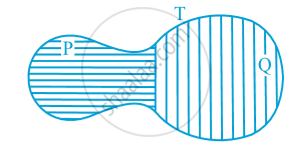Share

# Introduction to Areas of Parallelograms and Triangles

#### text

Now let us look at following fig.You may observe that planar region formed by figure T is made up of two planar regions formed by figures P and Q. You can easily see that
Area of figure T = Area of figure P + Area of figure Q.
You may denote the area of figure A as ar(A), area of figure B as ar(B), area of figure T as ar(T), and so on. Now you can say that area of a figure is a number (in some unit) associated with the part of the plane enclosed by the figure with the following  two properties:
(1) If A and B are two congruent figures, then ar(A) = ar(B);   and
(2) if a planar region formed by a figure T is made up of two non-overlapping planar regions formed by figures P and Q, then
ar(T) = ar(P) + ar(Q).

S